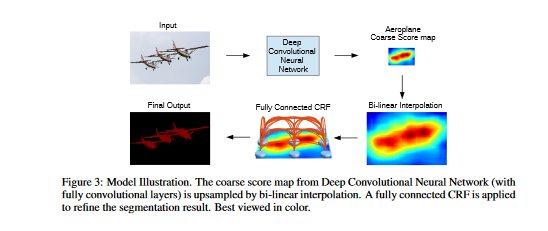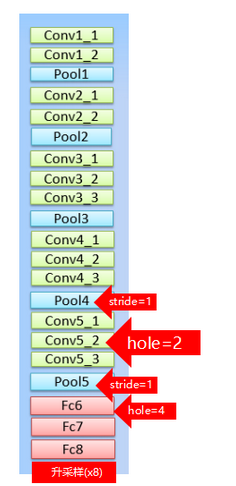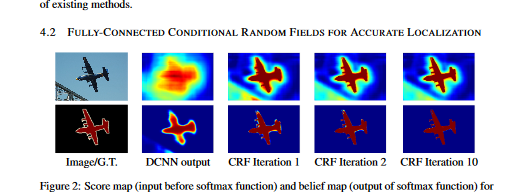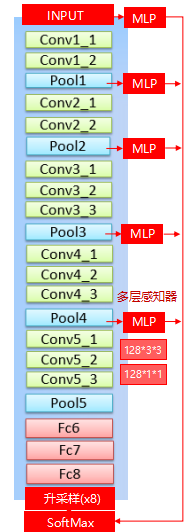# 论文原文¶

https://arxiv.org/pdf/1412.7062v3.pdf

# 模型结构¶DeepLab的BackBone依赖于VGG16，具体改造方法就是：

• 将最后的全连接层FC6,FC7,FC8改造成卷积层。
• pool4的stride由2变成1，则紧接着的conv5_1，conv5_2和conv5_3中hole size为2。
• 接着pool5由2变成1，则后面的fc6中的hole size为4。
• fc7，fc8为标准的卷积。
• 由于空洞卷积算法让feature map更加精细，因此网络直接采用插值上采样就能获得很好的结果，不用去学习上采样的参数了(FCN中采用了de-convolution)。

# 核心问题¶

## CRF¶CRF的计算公式为： $E(x)=\sum_i \theta_i(x_i)+\sum_{ij}\theta_{ij}(x_i,x_j)$ - x：对全局pixels的概率预测分布 - xi：其中一个pixel的概率预测分布 - θi：一元势函数 unary potential function $\theta_i(x_i):=-logP(x_i)$ - θij：二元势函数 $\theta_{ij}(x_i,x_j):=\sum_{m=1}^{K}w_mk^m(f_i,f_j)$ K : Kernel数量 w : 权重 本文采用高斯核，并且任意两个像素点都有此项，故称为fully connected CRFs。 $\theta_{ij}(x_i,x_j)=u(x_i,x_j)\sum_{m=1}^{K}w_mk^m(f_i,f_j), where u(x_i,x_j)=1 if x_i!=x_j$

## 多尺度预测¶

• 多尺寸预测，希望获得更好的边界信息。
• 引入：与FCN skip layer类似。
• 实现：
• 在输入图片与前四个max pooling后添加MLP（多层感知机,第一层是128个3×3卷积，第二层是128个1×1卷积），得到预测结果。
• 最终输出的特征映射送到模型的最后一层辅助预测，合起来模型最后的softmax层输入特征多了5×128=6405×128=640个通道。
• 效果不如dense CRF，但也有一定提高。最终模型是结合了Dense CRF与Multi-scale Prediction。 这里有个效果图如下（转自：https://blog.csdn.net/qq_31622015/article/details/90551107）：# 训练¶

DCNN模型采用预训练的VGG16，DCNN损失函数采用交叉熵损失函数。训练器采用SGD，batchsize设置为20。学习率初始为0.001，最后的分类层是0.01。每2000次迭代乘0.1。权重衰减为0.9的动量， 0.0005的衰减。 DeepLab由DCNN和CRF组成，训练策略是分段训练，即DCNN的输出是CRF的一元势函数，在训练CRF时是固定的。在对DCNN做了fine-tune后，对CRF做交叉验证。这里使用 ω2=3 和 σγ=3 在小的交叉验证集上寻找最佳的 ω1,σα,σβ。

# 代码实现¶

https://github.com/yilei0620/RGBD-Slam-Semantic-Seg-DeepLab/blob/master/slam_deepLab/model/test.prototxt

# 参考博客¶

https://blog.csdn.net/qq_31622015/article/details/90551107